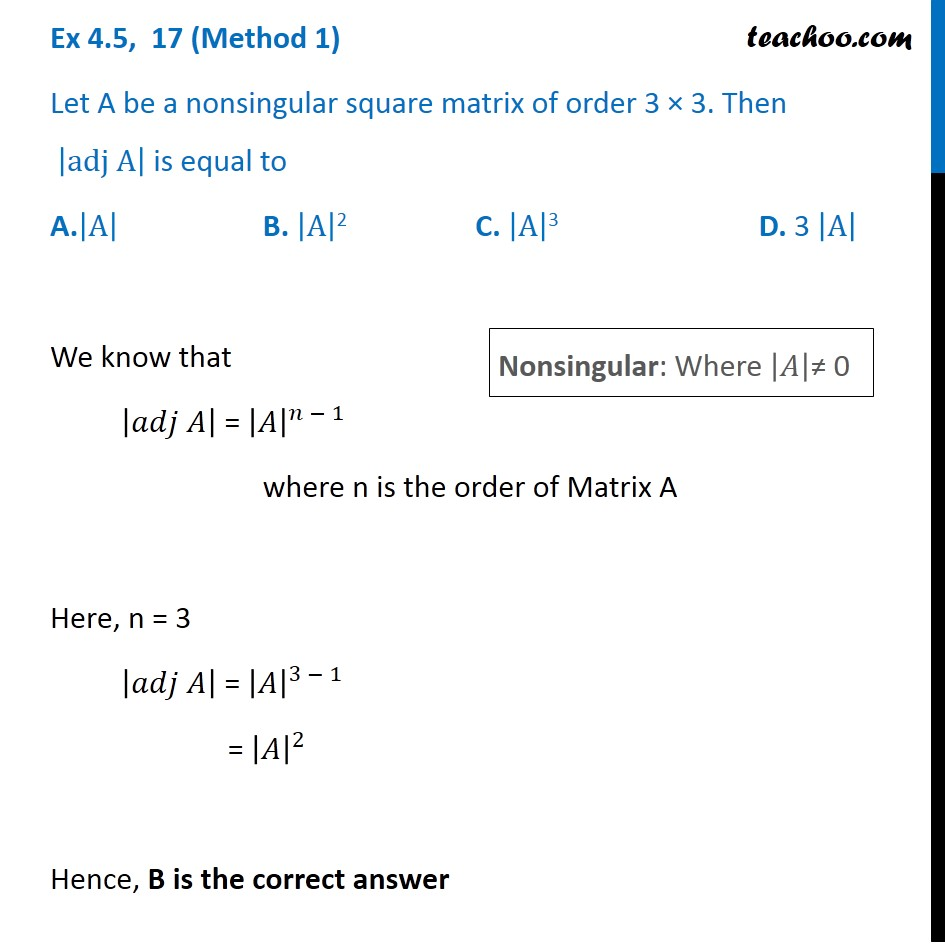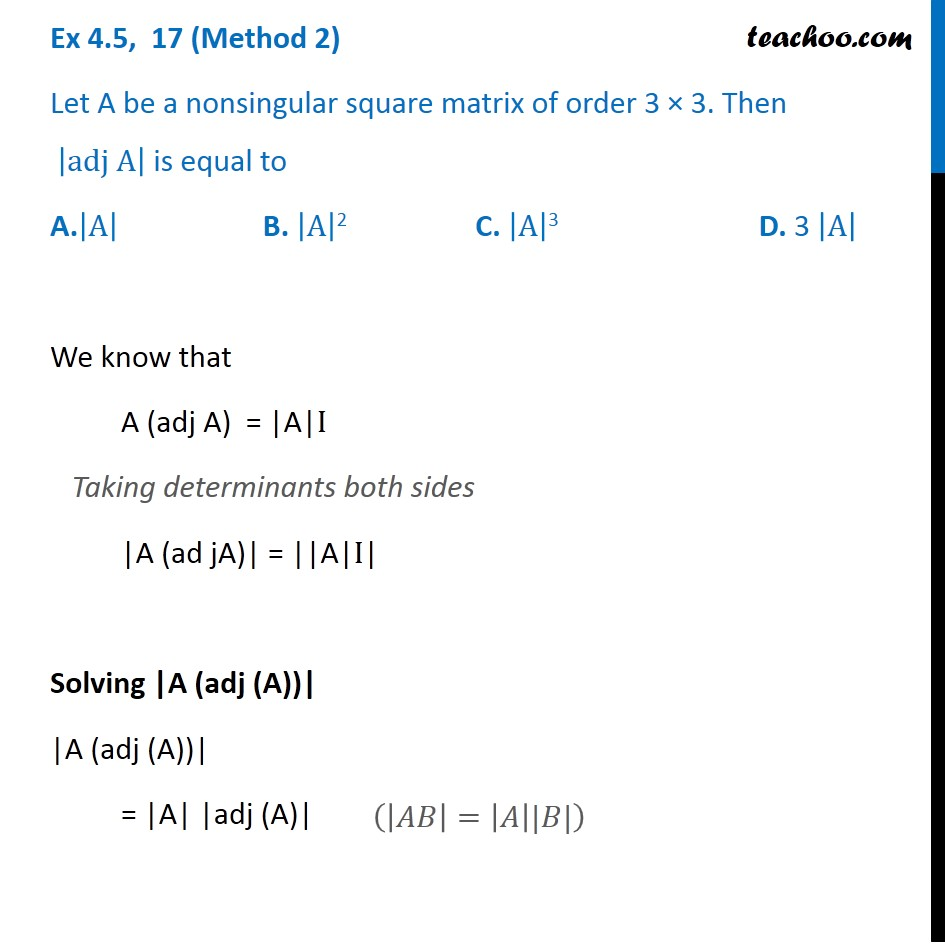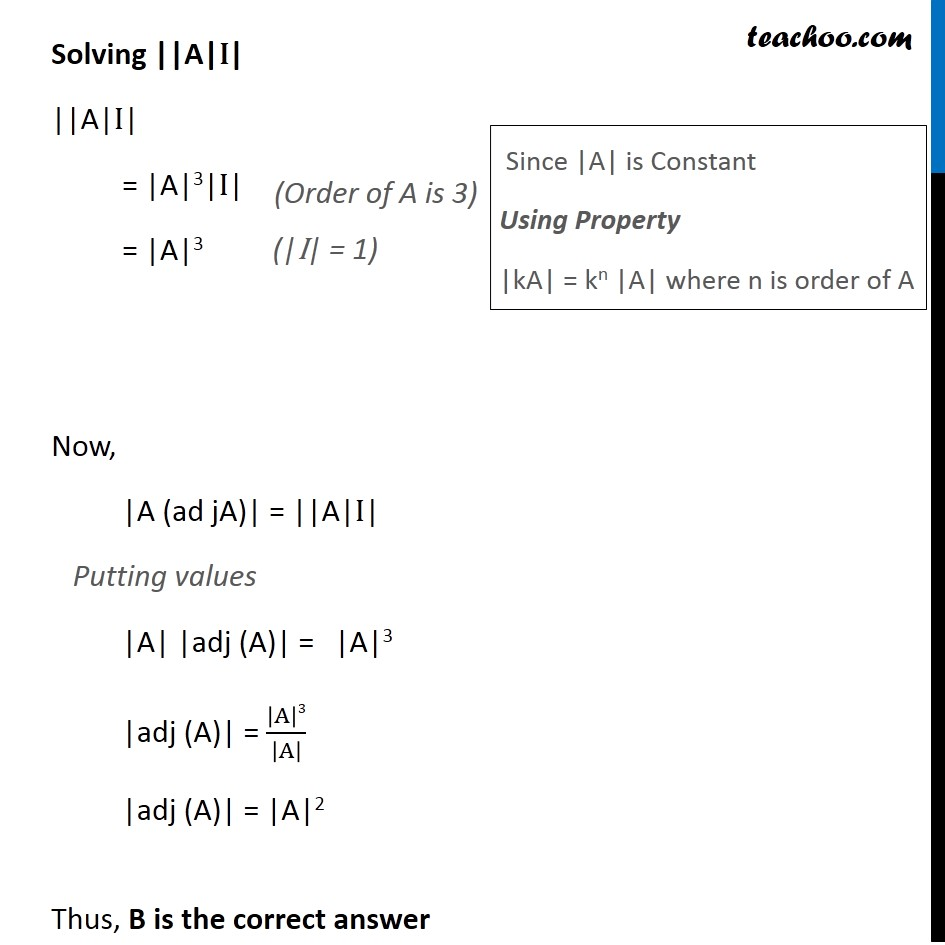Ex 4.5

Chapter 4 Class 12 Determinants
Serial order wiseSolve all your doubts with Teachoo Black (new monthly pack available now!)

### Transcript

Ex 4.5, 17 (Method 1) Let A be a nonsingular square matrix of order 3 × 3. Then |adj A| is equal to A. |A| B. |A|2 C. |A|3 D. 3 |A| We know that |𝑎𝑑𝑗 𝐴| = |𝐴|^(𝑛 − 1) where n is the order of Matrix A Here, n = 3 |𝑎𝑑𝑗 𝐴| = |𝐴|^(3 − 1) = |𝐴|^2 Hence, B is the correct answer Nonsingular: Where |𝐴|≠ 0 Ex 4.5, 17 (Method 2) Let A be a nonsingular square matrix of order 3 × 3. Then |adj A| is equal to A. |A| B. |A|2 C. |A|3 D. 3 |A| We know that A (adj A) = |A|I Taking determinants both sides |A (ad jA)| = ||A|I| Solving |A (adj (A))| |A (adj (A))| = |A| |adj (A)| (|𝐴𝐵|=|𝐴||𝐵|) Solving ||A|I| ||A|I| = |A|3|I| = |A|3 Now, |A (ad jA)| = ||A|I| Putting values |A| |adj (A)| = |A|3 |adj (A)| = |A|3/|A| |adj (A)| = |A|2 Thus, B is the correct answer Since |A| is Constant Using Property |kA| = kn |A| where n is order of A